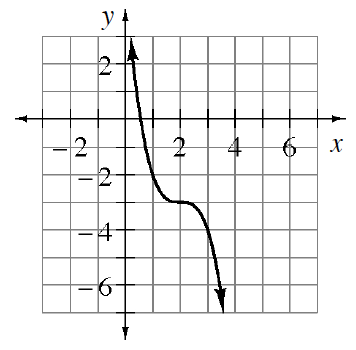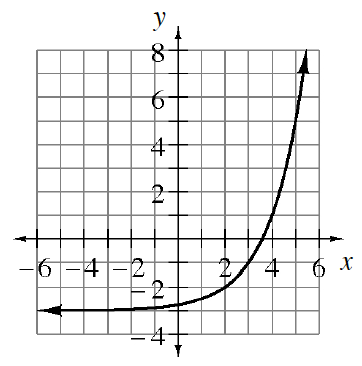### Home > PC3 > Chapter 5 > Lesson 5.1.3 > Problem5-34

5-34.

​ Write an equation for each function graphed below.

1.What parent function resembles this curve?

Where is the locator point?

What is the general equation of a cubic function?

Substitute the locator point for the horizontal and vertical shifts.

How should the equation show that the curve is reflected vertically?

A cubic function is a possible answer.

$(2,−3)$

$y=a(x−h)^3+k$

$y=a(x−2)^3−3$

$y=−(x−2)^3−3$

1.Follow a similar procedure as outlined in part (a).